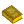## Logic

The formal mathematical study of the methods, structure, and validity of mathematical deduction and proof. Formal logic seeks to devise a complete, consistent formulation of mathematics such that propositions can be formally stated and proved using a small number of symbols with well-defined meanings. While this sounds like an admirable pursuit in principle, in practice the study of mathematical logic can rapidly become bogged down in pages of dense and unilluminating mathematical symbols, of which Whitehead and Russell's Principia Mathematica (1925) is perhaps the best (or worst) example.

A very simple form of logic is the study of ``Truth Tables'' and digital logic circuits in which one or more outputs depend on a combination of circuit elements (AND, NAND, OR, XOR, etc.; ``gates'') and the input values. In such a circuit, values at each point can take on values of only True (1) or False (0). de Morgan's Duality Law is a useful principle for the analysis and simplification of such circuits.

A generalization of this simple type of logic in which possible values are True, False, and ``undecided'' is called Three-Valued Logic. A further generalization called Fuzzy Logic treats ``truth'' as a continuous quantity ranging from 0 to 1.

See also Absorption Law, Alethic, Boolean Algebra, Boolean Connective, Bound, Caliban Puzzle, Contradiction Law, de Morgan's Duality Law, de Morgan's Laws, Deducible, Excluded Middle Law, Free, Fuzzy Logic, Gödel's Incompleteness Theorem, Khovanski's Theorem, Logical Paradox, Logos, Löwenheim-Skolem Theorem, Metamathematics, Model Theory, Quantifier, Sentence, Tarski's Theorem, Tautology, Three-Valued Logic, Topos, Truth Table, Turing Machine, Universal Statement, Universal Turing Machine, Venn Diagram, Wilkie's Theorem

ReferencesLogic

Adamowicz, Z. and Zbierski, P. Logic of Mathematics: A Modern Course of Classical Logic. New York: Wiley, 1997.

Bogomolny, A. ``Falsity Implies Anything.'' http://www.cut-the-knot.com/do_you_know/falsity.html.

Carnap, R. Introduction to Symbolic Logic and Its Applications. New York: Dover, 1958.

Church, A. Introduction to Mathematical Logic, Vol. 1. Princeton, NJ: Princeton University Press, 1996.

Gödel, K. On Formally Undecidable Propositions of Principia Mathematica and Related Systems. New York: Dover, 1992.

Jeffrey, R. C. Formal Logic: Its Scope and Limits. New York: McGraw-Hill, 1967.

Kac, M. and Ulam, S. M. Mathematics and Logic: Retrospect and Prospects. New York: Dover, 1992.

Kleene, S. C. Introduction to Metamathematics. Princeton, NJ: Van Nostrand, 1971.

Whitehead, A. N. and Russell, B. Principia Mathematica, 2nd ed. Cambridge, England: Cambridge University Press, 1962.